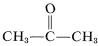# 10.3: The Reactivity of Multiple Carbon-Carbon Bonds

$$\newcommand{\vecs}{\overset { \rightharpoonup} {\mathbf{#1}} }$$ $$\newcommand{\vecd}{\overset{-\!-\!\rightharpoonup}{\vphantom{a}\smash {#1}}}$$$$\newcommand{\id}{\mathrm{id}}$$ $$\newcommand{\Span}{\mathrm{span}}$$ $$\newcommand{\kernel}{\mathrm{null}\,}$$ $$\newcommand{\range}{\mathrm{range}\,}$$ $$\newcommand{\RealPart}{\mathrm{Re}}$$ $$\newcommand{\ImaginaryPart}{\mathrm{Im}}$$ $$\newcommand{\Argument}{\mathrm{Arg}}$$ $$\newcommand{\norm}{\| #1 \|}$$ $$\newcommand{\inner}{\langle #1, #2 \rangle}$$ $$\newcommand{\Span}{\mathrm{span}}$$ $$\newcommand{\id}{\mathrm{id}}$$ $$\newcommand{\Span}{\mathrm{span}}$$ $$\newcommand{\kernel}{\mathrm{null}\,}$$ $$\newcommand{\range}{\mathrm{range}\,}$$ $$\newcommand{\RealPart}{\mathrm{Re}}$$ $$\newcommand{\ImaginaryPart}{\mathrm{Im}}$$ $$\newcommand{\Argument}{\mathrm{Arg}}$$ $$\newcommand{\norm}{\| #1 \|}$$ $$\newcommand{\inner}{\langle #1, #2 \rangle}$$ $$\newcommand{\Span}{\mathrm{span}}$$$$\newcommand{\AA}{\unicode[.8,0]{x212B}}$$

In the early days of organic chemistry, alkenes were described as "unsaturated" because, in contrast to the "saturated" alkanes, they were found to react readily with substances such as halogens, hydrogen halides, oxidizing agents, and so on. Therefore, the "chemical affinity" of alkenes was regarded as unsatisfied or "unsaturated". (Also see Section 1-1I.)

One reason alkenes and alkynes react more readily than alkanes is because the carbon-carbon bonds of a multiple bond are individually weaker than normal carbon-carbon single bonds. Consider the bond energies involved. According to Table 4-3, the strengths of carbon-carbon single, double, and triple bonds are $$83$$, $$146$$, and $$200 \: \text{kcal}$$, respectively. From these values we can calculate that cleavage of one-half of a carbon-carbon double bond should require $$63 \: \text{kcal}$$ and cleavage of one-third of a carbon-carbon triple bond should require $$54 \: \text{kcal}$$:As a result, addition reactions to multiple bonds are expected to be about $$20$$-$$30 \: \text{kcal}$$ more exothermic than the corresponding cleavage reactions of carbon-carbon single bonds, as estimated here for reaction with bromine:

The substantial difference in the heats of reaction of ethane, ethene, and ethyne with bromine is reflected in a very important practical consideration in handling ethyne (acetylene), namely its thermodynamic stability relative to solid carbon and hydrogen gas. Unlike ethane, both ethene and ethyne can be shown from bond energies to be unstable with respect to formation of solid carbon and gaseous hydrogen:

Although this does not seem to offer particular problems with ethene, an explosive decomposition of ethyne to carbon and hydrogen may occur if the gas is compressed to $$10$$-$$20 \: \text{kg cm}^{-2}$$. Even liquid ethyne (bp \sim 83^\text{o}\)) must be handled with care. Ethyne is not used commercially under pressure unless it is mixed with an inert gas and handled in rugged equipment. Ethyne burns with pure oxygen to give a very hot flame that is widely used for welding. For this purpose, the gas is dissolved under about $$15 \: \text{kg cm}^{-2}$$ in 2-propanone (acetone,, bp $$56.5^\text{o}$$) and contained in cylinders packed with diatomaceous earth.

Why is ethyne so much less stable than ethene or ethane? First, $$\ce{C-C}$$ bonds are not as strong as $$\ce{C-H}$$ bonds. Therefore a gain in stability usually is to be expected when $$\ce{C-H}$$ bonds are made at the expense of $$\ce{C-C}$$ bonds; ethene and ethane each have more $$\ce{C-H}$$ bonds than ethyne has. Second, ethyne has six electrons held between the two carbons and these electrons experience considerable mutual interelectronic repulsion. This accounts for the fact that the average $$\ce{C-C}$$ bond strength for the triple bond of an alkyne is $$200/3 = 67 \: \text{kcal}$$, compared to $$146/2 = 73 \: \text{kcal}$$ for the double bond of an alkene and $$83 \: \text{kcal}$$ for a normal single bond of an alkane.

## References

John D. Robert and Marjorie C. Caserio (1977) Basic Principles of Organic Chemistry, second edition. W. A. Benjamin, Inc. , Menlo Park, CA. ISBN 0-8053-8329-8. This content is copyrighted under the following conditions, "You are granted permission for individual, educational, research and non-commercial reproduction, distribution, display and performance of this work in any format."